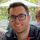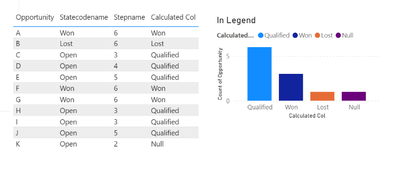cancel
Showing results for
Did you mean:## Conditional column

Hello!

Let's see if anyone can help me. I tell you:

I have a column named STEPNAME and another STATECODENAME.

I need to create a conditional column that meets the following:

1. [Statecodename] = "Won" ----> "Earned"

2. [Statecodename] = "Lost" ----> "Lost"

3. [Statecodename] = "Open" and [Stepname] = "3" ----> "Qualified"

4. [Statecodename] = "Open" and [Stepname] = "4" ----> "Qualified"

5. [Statecodename] = "Open" and [Stepname] = "5"----> "Qualified"

I had tried this formula, but the "Qualified" returns them to me as null and it is not correct:

else if [statecodename] = "Perdida" then "Perdida"

else if [statecodename] = "Abierta" & [Stepname] = "3" then "Cualificada"

else if [statecodename] = "Abierta" & [Stepname] = "4" then "Cualificada"

else if [statecodename] = "Abierta" & [Stepname] = "5" then "Cualificada"

else null)

I need this conditional column to put as a legend on a chart.

How can I make a formula that fulfills all this and that I get in a column those that are WON, LOST and QUALIFIED?

1 ACCEPTED SOLUTIONSuper User

Hi,

The following calculated column logic works:

`Calculated Col = IF( Statecode[Statecodename] = "Won", "Won",  IF(Statecode[Statecodename] = "Lost", "Lost",  IF(Statecode[Statecodename] = "Open" && Statecode[Stepname] = 3, "Qualified",  IF(Statecode[Statecodename] = "Open" && Statecode[Stepname] = 4, "Qualified", IF(Statecode[Statecodename] = "Open" && Statecode[Stepname] = 5, "Qualified", "Null")))))`

Below is the returned results with some dummy data.2 REPLIES 2Community Support

I think johncolley's answer is correct, here is a more concise method by the way.

``````Calculated Col =
SWITCH (
Statecode[Statecodename],
"Won", "Won",
"Lost", "Lost",
"Open",
IF ( Statecode[Stepname] = 3 || [Stepname] = 4 || [Stepname] = 5, "Qualified" )
)
``````

Best Regards,
Community Support Team _ Yalan Wu
If this post helps, then please consider Accept it as the solution to help the other members find it more quickly.Super User

Hi,

The following calculated column logic works:

`Calculated Col = IF( Statecode[Statecodename] = "Won", "Won",  IF(Statecode[Statecodename] = "Lost", "Lost",  IF(Statecode[Statecodename] = "Open" && Statecode[Stepname] = 3, "Qualified",  IF(Statecode[Statecodename] = "Open" && Statecode[Stepname] = 4, "Qualified", IF(Statecode[Statecodename] = "Open" && Statecode[Stepname] = 5, "Qualified", "Null")))))`

Below is the returned results with some dummy data.